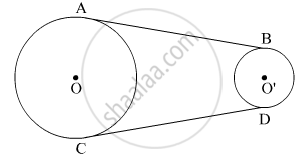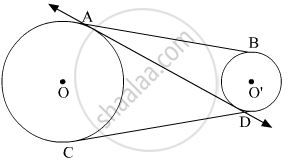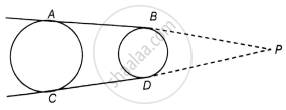# In the Figure, Ab and Cd Are Common Tangents to Two Circles of Unequal Radi. Prove that Ab = Cd - Mathematics

Sum

In the figure, AB and CD are common tangents to two circles of unequal radi. Prove that AB = CD#### Solution 1

Construct a line passing through ADNow, AD and CD are tangents to the circle with centre O from the external point D.
So, AD = CD    (Tangents drawn from an external point to a circle are equal.)         .....(1)

Also, AB and AD are the tangents to the circle with centre O' from the external point A.
So, AD = AB    (Tangents drawn from an external point to a circle are equal.)          .....(2)

From (1) and (2)

AB = CD

Hence Proved.

#### Solution 2

Given: AB and CD are two common tangents to two circles of unequal radii.

To Prove: AB = CDConstruction: Produce AB and CD, to intersect at P.

Proof: Consider the circle with greater radius.

AP = CP  ......[Tangents drawn from an external point to a circle are equal] 

Also, Consider the circle with smaller radius.

BP = BD   ......[Tangents drawn from an external point to a circle are equal] 

Substract  from , we get

AP - BP = CP – BD

AB = CD

Hence Proved .

Concept: Tangent to a Circle
Is there an error in this question or solution?

#### APPEARS IN

NCERT Mathematics Exemplar Class 10
Chapter 9 Circles
Exercise 9.3 | Q 5 | Page 107
Share